80s toys - Atari. I still have

HARGA
Update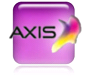AX5 = 4.960
AX10 = 9.910
AX25 = 24.760
AX50 = 49.510
AX100 = 99.010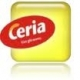CE5 = 4.760
CE10 = 9.510
CE20 = 19.010
CE50 = 47.510
CE100 = 95.010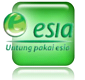ES1 = 1.110
ES5 = 5.160
ES10 = 10.160
ES11 = 11.175
ES15 = 15.210
ES20 = 20.260
ES25 = 25.110
ES50 = 49.010
ES100 = 96.260F5 = 4.960
F10 = 9.710
F20 = 19.360
F50 = 48.260
F100 = 96.260FR10 = 9.710
FR25 = 24.210
FR50 = 48.160
FR100 = 95.860HP5 = 5.060
HP10 = 9.910
HP25 = 24.510
HP50 = 48.510
HP100 = 97.010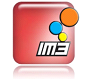I5 = 4.985
IS5 = 4.985
IG5 = 4.985
IG10 = 9.985
I10 = 9.985
I2 = 2.060
I25 = 24.910
I50 = 49.560
I100 = 98.810
IM3/MENTARI LINTAS
IL5 = 5.110
ILS5 = 4.985
IS10 = 9.985
IS25 = 25.060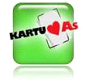SJ5 = 5.085
SJ10 = 10.135
SJ20 = 20.010
SJ50 = 49.090
SJ100 = Rp97.510
TELKOMSEL PROMO
SP5 = 5.335
SP10 = 10.110
SP20 = 20.010
TELKOMSEL NASIONAL (S/AS)
S5 = 5.360
S10 = 10.110
S20 = 20.035
S25 = 25.310
S50 = 49.240
S100 = 97.510SMA5 = 4.935
SMA10 = 9.835
SMA20 = 19.560
SMA50 = 49.010
SMA100 = 97.510ST5 = 5.160
ST10 = 10.160
ST25 = 24.910
ST50 = 49.560
ST100 = 99.010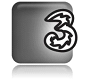T1 = 1.010
T3 = 3.010
T5 = 5.010
T10 = 9.910
T30 = 29.710
T50 = 49.260
T100 = 98.510XB1 = 1.135
XB5 = 5.285
XB10 = 10.210
XB25 = 25.110
XB50 = 50.060
XB100 = 100.060

XL ALTERNATIF
X5 = 5.310
X10 = 10.285

IM2
MM5 = 4.985
MM10 = 9.985
MM25 = 25.010
MM50 = 50.010
MM100 = 99.410